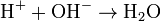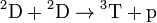# Chemical symbol

## Contents

Chemical symbols are the international standard way to denote chemical elements. In particular, they are used in chemical formulas to describe the composition and structure of molecules, and in reaction formulas. A chemical symbol consists of one or two letters — the initial letter of its scientific name in uppercase which, in most cases, is followed by a suitable lowercase letter from the name. (Sometimes, on a temporary basis, for new artificial elements initially 3-letter symbols are used.)

Well-known examples of one-letter symbols are H for hydrogen and O for oxygen, Ca is the two-letter symbol for calcium. For most elements the symbol fits its English name because this name is also derived from the scientific name (which usually is of Greek or Latin origin). However, in a few cases there is no relation between the English word and its symbol. For instance, Fe (derived from Latin "ferrum") is the symbol for iron.
Element number 112 has the symbol Uub, for its temporary name Ununbium (which is derived from Latin for one-one-two).

For a complete list of all chemical symbols see the alphabetical list.

In addition, subscripts and superscripts attached to the basic symbol are used to carry additional information.

##  Compounds

The number of atoms in a molecule is indicated by a subscript (on the right). For example, O2 is oxygen, and O3 is ozone.

##  Ions

An ion of an element is indicated by a superscript, where + and - stand for positive and negative charge, respectively. For example, H+ means a hydrogen ion, and Ca2+ a calcium ion (with two electrons missing).
The ion H+ of hydrogen is a proton which — in atomic reactions — is also indicated as p. (Similarly, a neutron is indicated by n.)

##  Isotopes

In atom physics, isotopes of an element are distinguished by adding the atomic mass as a superscript (usually, but not always, attached to the left of the symbol).
The isotopes of hydrogen have symbols of their own: 1H is hydrogen H, 2H is deuterium D, and 3H is tritium T.

For convenience, sometimes the atomic number — which is already implied by the chemical symbol &mdash is added as subscript on the left.

##  Examples

A simple example for a chemical reaction formula is$\rm 2 H_2 + O_2 \to 2 H_2 O$

which states that hydrogen and oxygen can react and produce water.

A hydrogen ion and and a OH-group combine to water:$\rm H^+ + OH^- \to H_2 O$

In an atomic fusion reaction, two deuterium atoms can either combine to a tritium atom and emit a proton:$\rm {}^2D + {}^2D \to {}^3T + p$

or to a helium isotope and emit a neutron:$\rm {}^2_1D + {}^2_1D \to {}^3_2He + n$Some content on this page may previously have appeared on Citizendium.